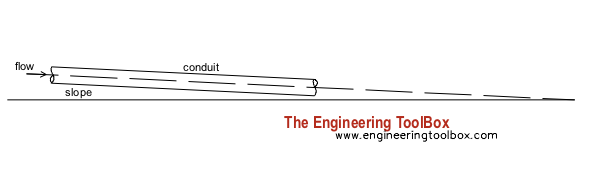Engineering ToolBox - Resources, Tools and Basic Information for Engineering and Design of Technical Applications!

# Chezys Conduit Flow Equation

## Calculating volume flow and velcity in open conduits.The Chezys equation can be used to mean flow velocity in conduits:

v = C (Rh S)1/2                           (1)

where

v = mean velocity (m/s, ft/s)

C = Chezys roughness and conduit coefficient (m1/2/s)

Rh = hydraulic radius of the conduit (m, ft)

S = slope of the conduit (m/m, ft/ft)

In general the Chezy coefficient - C - is a function of the flow Reynolds Number - Re - and the relative roughness - ε/R - of the channel. ε is the characteristic height of the roughness elements on the channel boundary.

The Manning empirical relationship is one way to estimate the roughness coefficient C:

C = (1 / n) Rh1/6

where

n = Manning coefficient of roughness

For concrete walls in conduits it's common to use C = 50 (m1/2/s).

### Example - Flow in a Concrete Conduit

A rectangular 1 (m) x 1 (m) concrete conduit with slope 1/100 (m/m) is half filled with water.

The cross sectional area of the filled conduit can be calculated as

A = 0.5 * (1 m) * (1 m)

= 0.5 m2

The wetted perimeter of the filled conduit can be calculated as

P = 2 * 0.5 * (1 m) + (1 m)

= 2 m

The hydraulic radius can be calculated as

Rh = A / P

= (0.5 m2) / (2 m)

= 0.25 m

The velocity in the flow can be calculated using eq. (1) as

v = (50 m1/2/s) ((0.25 m) (1/100 m/m))1/2

= 2.5 m/s

The volume flow can be calculated as

q = A v

= (0.5 m2) (2.5 m/s)

= 1.25 m3/s

## Related Topics

• ### Fluid Mechanics

The study of fluids - liquids and gases. Involving velocity, pressure, density and temperature as functions of space and time.

## Related Documents

• ### Flow Section Channels - Geometric Relationships

Geometric relationships like area, wetted perimeter and hydraulic diameter.
• ### Fluid Mechanics - Imperial and SI Units vs. Dimensions

Imperial (USCS) and SI dimensions and units terminology in fluid mechanics.
• ### Manning's Formula and Gravity Flow

Calculate cross-sectional average velocity flow in open channels.

• ### Surface Roughness vs. Mechanical Processing

Mechanical production methods and their surface roughness.

## Engineering ToolBox - SketchUp Extension - Online 3D modeling!

Add standard and customized parametric components - like flange beams, lumbers, piping, stairs and more - to your Sketchup model with the Engineering ToolBox - SketchUp Extension - enabled for use with older versions of the amazing SketchUp Make and the newer "up to date" SketchUp Pro . Add the Engineering ToolBox extension to your SketchUp Make/Pro from the Extension Warehouse !

We don't collect information from our users. More about

## Citation

• The Engineering ToolBox (2005). Chezys Conduit Flow Equation. [online] Available at: https://www.engineeringtoolbox.com/chezy-formula-d_964.html [Accessed Day Month Year].

Modify the access date according your visit.

9.27.11